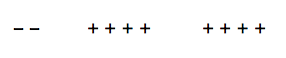Home > CC1MN > Chapter cc13 > Lesson cc13.2.2 > Problem3-106

3-106.

Find the missing value in each number sentence. Homework Help ✎

1. $4 - 3.5 + 3.5 - 1 = \text {_____}$

• Since $−3.5 + 3.5 = 0$, this number sentence can be written equivalently as $4 − 1$.

1. $- 2 + 4 + 4 = \text{_____}$

• Try using integer tiles.1. $5 \frac { 1 } { 3 } - 7 - 5 \frac { 1 } { 3 } = \text{_____}$

• Like in part (a), there are two terms which sum to 0 in this number sentence.

$−7$